# Smooth RGB Image Using Min Max Curvature Flow#

## Synopsis#

Smooth an RGB image using min/max curvature flow.

## Code#

### C++#

#include "itkImageAdaptor.h"
#include "itkImageRegionIterator.h"
#include "itkMinMaxCurvatureFlowImageFilter.h"
#include "itkComposeImageFilter.h"
#include "itkImage.h"
#include "itkRGBPixel.h"

#include <sstream>

#ifdef ENABLE_QUICKVIEW
#  include "QuickView.h"
#endif

int
main(int argc, char * argv[])
{
// Verify command line arguments
if (argc < 2)
{
std::cerr << "Usage: " << std::endl;
std::cerr << argv << " InputImageFile [iterations]" << std::endl;
return EXIT_FAILURE;
}

int iterations = 5;
if (argc > 2)
{
std::stringstream ss(argv);
ss >> iterations;
}
std::string inputFileName = argv;

// Setup types
using ComponentType = float;
using PixelType = itk::RGBPixel<ComponentType>;
using RGBImageType = itk::Image<PixelType, 2>;
using ImageType = itk::Image<ComponentType, 2>;
using ComposeType = itk::ComposeImageFilter<ImageType, RGBImageType>;

// Run the filter for each component

auto rCurvatureFilter = CurvatureFlowType::New();
rCurvatureFilter->SetNumberOfIterations(iterations);
rCurvatureFilter->Update();

auto gCurvatureFilter = CurvatureFlowType::New();
gCurvatureFilter->SetNumberOfIterations(iterations);
gCurvatureFilter->Update();

auto bCurvatureFilter = CurvatureFlowType::New();
bCurvatureFilter->SetNumberOfIterations(iterations);
bCurvatureFilter->Update();

// compose an RGB image from the three filtered images

auto compose = ComposeType::New();
compose->SetInput1(rCurvatureFilter->GetOutput());
compose->SetInput2(gCurvatureFilter->GetOutput());
compose->SetInput3(bCurvatureFilter->GetOutput());

#ifdef ENABLE_QUICKVIEW
QuickView viewer;

std::stringstream desc;
desc << "MinMaxCurvatureFlow iterations = " << iterations;

viewer.Visualize();
#endif

return EXIT_SUCCESS;
}


## Classes demonstrated#

template<typename TInputImage, typename TOutputImage>
class MinMaxCurvatureFlowImageFilter : public itk::CurvatureFlowImageFilter<TInputImage, TOutputImage>

Denoise an image using min/max curvature flow.

MinMaxCurvatureFlowImageFilter implements a curvature driven image denoising algorithm. Iso-brightness contours in the grayscale input image are viewed as a level set. The level set is then evolved using a curvature-based speed function: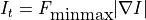where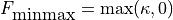if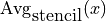is less than or equal to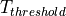and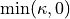, otherwise.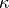is the mean curvature of the iso-brightness contour at point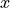.

In min/max curvature flow, movement is turned on or off depending on the scale of the noise one wants to remove. Switching depends on the average image value of a region of radius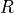around each point. The choice of, the stencil radius, governs the scale of the noise to be removed.

The threshold valueis the average intensity obtained in the direction perpendicular to the gradient at pointat the extrema of the local neighborhood.

This filter make use of the multi-threaded finite difference solver hierarchy. Updates are computed using a MinMaxCurvatureFlowFunction object. A zero flux Neumann boundary condition is used when computing derivatives near the data boundary.

Reference: “Level Set Methods and Fast Marching Methods”, J.A. Sethian, Cambridge Press, Chapter 16, Second edition, 1999.

Warning

This filter assumes that the input and output types have the same dimensions. This filter also requires that the output image pixels are of a real type. This filter works for any dimensional images, however for dimensions greater than 3D, an expensive brute-force search is used to compute the local threshold.

See

MinMaxCurvatureFlowFunction

See

CurvatureFlowImageFilter

See

BinaryMinMaxCurvatureFlowImageFilter

ITK Sphinx Examples:

Subclassed by itk::BinaryMinMaxCurvatureFlowImageFilter< TInputImage, TOutputImage >